Definitions
 Home | Definitions | Properties | Interpretations | Applications | Gambling | Software | Books

Experiments, events

An experiment is a type of action that generates events. An example of an experiment is rolling the die. The performance of an experiment is called test or trial. The experiments generate outcomes (results). An experiment can have more than one outcome, while a test can have only one outcome.

Example: The experiment of rolling the die can have six outcomes (namely the numbers from 1 to 6 inscribed on die’s sides).

An event is an arbitrary set of pre-established outcomes of an experiment. An event can happen (occur) or not, as result of an experiment. If e is the outcome of a test and A an event related to the respective experiment, we say that A happens if e belongs to A and A does not happen if e does not belong to A.

Example:  In the roll of a die, the set of all possible outcomes is {1, 2, 3, 4, 5, 6}. Some events are: A = {1, 3, 5} (uneven  number), B = {1, 2, 3, 4} (less than the number 5), C = {2, 4, 6} (even number). If rolling a 3, events A and B occur.

Denote by Ω the set of all possible outcomes of an experiment and by P (Ω) the set of all parts of Ω. Set Ω is called the set of outcomes or the sample space of the experiment. The random events are elements of P (Ω).

Operations and relations between events

On the set Σ of the events associated with an experiment, we can introduce three operations that correspond to the logical operations or, and, non. Let A and B be events from Σ.
a)  A or B is the event that occurs if, and only if, one of the events A or B occurs. This event is denoted byand is called the union or disjunction of events A and B.
b)  A and B is the event that occurs if, and only if, both events A and B occur. This event is denoted by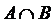and is called the intersection or conjunction of events A and B.
c)  non A is the event that occurs if, and only if, event A does not occur. This event is called the complement or opposite of A and is denoted by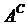.

If  A and B cannot occur simultaneously, we say that A and B are incompatible or mutually exclusive events and denote it by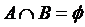. If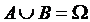, we say that A and B are collectively exhaustive.

In the set Σ of events associated with a certain experiment, two events with special significance exist, namely, eventand event. The first consists of the occurrence of event A or the occurrence of event, which obviously always happens; It is natural to call Ω the sure event. Event ϕ consists of the occurrence of event A and the occurrence of event, which can never happen. This event is called the impossible event.

Let A and B be events from Σ. We say that event A implies event B and write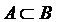, if, when A occurs, B necessarily occurs. If we haveand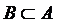, we say that events A and B are equivalent and write A = B.

Definition:  An event A fromis said to be compound if there exist two events B and C from, such thatand. Otherwise, the event A is said to be elementary.

Example: In the experiment of rolling the die:
– Event {3, 5} is compound, because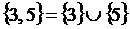;
– Event {1, 2, 4} is compound, because;
– Events {1}, {2}, {3}, {4}, {5}, {6} are elementary.

Boole algebras

Definition:  Call Boole algebra a nonempty set A, with operations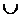,,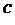defined and meeting the following axioms:
1.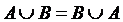;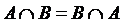(commutativity);
2.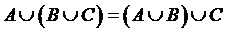;(associativity);
3.(absorption);
4.;(distributivity);
5.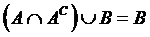;(complementarity),
for anyA.

Axiom: The set of events associated to an experiment is a Boole algebra.

Example: The set of parts P (Ω) of a nonempty set, with union, intersection and complement (related to Ω) operations, gets a Boole algebra structure.

Definition:  The Boole algebra of the events associated to an experiment is called the field of events of that experiment.

So, the field of events is a set of parts of Ω, structured as an algebra of events Σ, and is denoted by {Ω, Σ}.
Therefore, the events can be represented as sets and the denotations of their operations as from set theory are entirely justified.

We should retain:
● the events are mathematical objects assimilated with sets;
● the operations between sets also hold for events;
● the set of events associated to an experiment has a Boolean structure with respect to the operations and, or and non;
● any experiment has a sample space and a field of events attached.

Read the next definitions.

 Sources The book UNDERSTANDING AND CALCULATING THE ODDS: Probability Theory Basics and Calculus Guide for Beginners, with Applications in Games of Chance and Everyday Life is addressed to non-mathematicians and builds a clear image of the probability concept by reconstructing its mathematical definition step by step through its constituent notions, starting with fundamental notions like sets, functions, convergence and measure theory basics. You may find it in the Books section with a free sample.Software - Books - Contact us